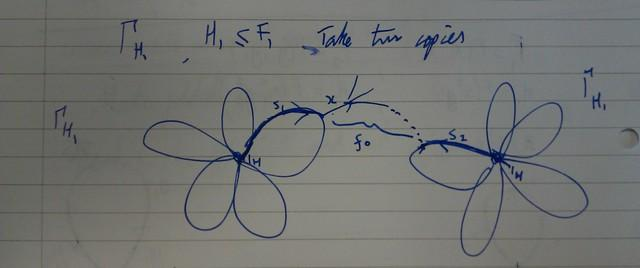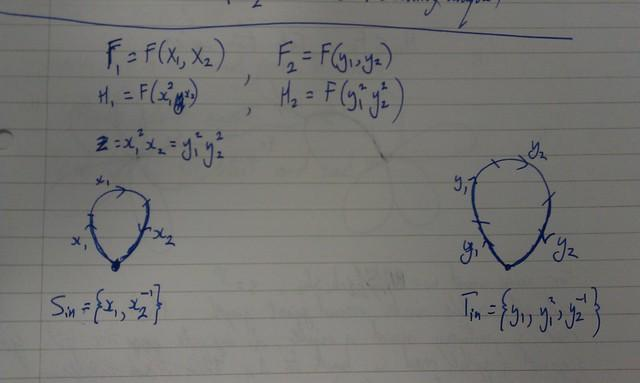# Double coset normal form for FPAs

Liza Frenkel is visiting the department, and she wants me to write an algorithm to compute double coset representation normal forms for free products with amalgamation.

Here are some notes I typed up in write maths, see maths as she and Andrew talked. I’m just putting them up here so I don’t lose them.

Suppose:
$$F_{1}$$, $$F_{2}$$ free groups (finitely generated by $$X$$ and $$Y$$, respectively)
$$H_1 \leq F_1$$, $$H_2 \leq F_2$$
$$\exists$$ an isomorphism $$\phi: H_1 \rightarrow H_2$$
i.e. we have a finite generating set (in fact a basis) $$\{h_1, \dots, h_n \}$$ for $$H_{1}$$ (and$$\{h_1′, \dots, h_n’\}$$ for $$H_{2}$$) and generating sets $$X,Y$$ for $$F_{1}$$ and $$F_{2}$$

Consider a group generated by $$z_1, \dots, z_m$$, with
$$z_1 = h_1(x_1, \dots, x_n)=h_1′(y_1,\dots,y_r), \dots, z_m = h_m(x_1,\dots,x_n) = h_m'(y_1,\dots,y_r)$$
$$G = F_1 \underset{H_1=H_2}{\ast} F_2$$ has presentation $$\langle X,Y | h_i = h_i’, i=1 \dots m\rangle$$
Choose a set $$S \subseteq F$$ such that $$F_1 = \displaystyle{\bigcup_{s \in S} H_1sH_1}$$
(we are assuming $$S$$ is infinite)
every element $$w$$ of $$F_{1}$$ is equal to $$w = h_{1} sh_{2}$$, for unique $$s \in S$$,
$$h_1,h_2 \in H_1$$
can do the same for $$F_2 = \displaystyle{\bigcup_{t \in T}} H_2tH_2$$

Let $$g \in G$$.
A word $$w$$ representing $$g$$ is in normal form if $$w = h_{i_1}(z)p_1h_{i_2}(z)p_2 \dots h_{i_k}(z)p_kh_{i_{k+1}}(z)$$, with $$p_i \in S$$, $$i = 1 \dots k$$.

Suppose $$g = g_1g_2 \dots g_k$$. Rewrite using double coset representatives
$$g = h_1(X)s_1h_2(Y)t_2h_3(X) \dots$$

$$g$$ is in reduced form if $$g = g_1 \dots g_k$$ and
$$k=1 \Rightarrow$$ $$g \in F_1$$ or $$g \in F_2$$
$$k \gt 1 \Rightarrow g_i \in F_1 \backslash H_1$$ or $$g_i \in F_2 \backslash H_2$$ and $$g_{i}$$, $$g_{i+1}$$ belong to different factors

Let $$\Gamma_{H_1}$$ be a folded subgroup graph of $$H_{1}$$. Take two copies.For some word $$w = h_{1} sh_{2}$$, the question is how to find $$s$$.

Algorithm:
Read all possible loops round first graph, to get $$h_{1}$$.
Keep reading letters round the graph until there is no edge corresponding to the current letter. Call this $$s_{1}$$.
Start at the other end, reading loops to get $$h_{2}$$, and then maximal $$s_{2}$$ partway round a loop.
Then either there are no letters left to look at, and $$s = s_{1} s_{2}$$, or some bit $$f_{0}$$ in the middle, and $$s = s_{1} f_{0} s_{2}$$.

We want $$h_1,h_2,s$$ to all have maximal length.

Examplei) \begin{align} g &= x_1^3x_2^2y_1y_2 \\ &= (x_1^2)(x_1x_2)(x_2^{-1})^{-1} \cdot (y_1)(y_2^{-1})^{-1}\end{align}

## Membership problem

\begin{align} G &= F_1 \underset{H_1=H_2}{\ast}F_2 \\ H_3 &= \langle c_1, \dots, c_l \rangle \leqslant G\end{align}
Can write the $$c_{i}$$ in normal form.
Question: Given $$w \in G$$ in normal form, is $$w \in H_3$$?

Constructing a folded graph which makes use of the normal form is tricky.